# Quantitative Aptitude Quiz For IBPS RRB PO, Clerk Prelims 2021- 23rd June

Q1. There are two vessel A and B containing mixture of milk and water in the ratio 5 : x and 8 : 15 respectively. Vessel A contains 48 L of mixture. 12 L of mixture from vessel A is replaced by 23 liter of mixture from vessel B. Final ratio of milk to total mixture in vessel A become 23 : 59. Find value of x.
(a) 7
(b) 6
(c) 5
(d) 3
(e) 2

Q2. Veer, Sameer and Neeraj invested their amount on simple interest in the ratio of 4 : 5 : 8 respectively. Rate of interest for Veer, Sameer and Neeraj is 12.5%, 10% and 20% respectively. After two years of investment Veer, Sameer and Neeraj joined a business with the amount obtained from interest only for 12 months, 18 month and 15 months respectively. If total profit from business is Rs 8450, then find the difference between profit shares of Neeraj and Sameer?
(a) 3625 Rs.
(b) 3650 Rs.
(c) 3850 Rs.
(d) 3750 Rs.
(e) 3250 Rs.

Q3. There are three vessels P , Q and R, Vessel P and Q filled with mixture of milk and Water in the ratio of 5 : 4 and 5 : 3 respectively. 25% of mixture from vessel P taken out and mixed in vessel R, which contains 45 lpure milk . If ratio of milk and water in vessel R becomes 7 : 2 and initial quantity of mixture in vessel Q is 20 l less than that of mixture in vessel P. then find the quantity of milk in vessel Q?
(a) 180 liters
(b) 120 liters
(c) 80 liters
(d) 100 liters
(e) 140 liters

Q4. In vessel X, mixture of water and wine is in the ratio of 3 : 4. 56 liters of mixture from vessel X is taken out and poured in another vessel Y which contains 48 liter of water only. If quantity of wine in vessel Y is one-fourth of the initial quantity of wine in vessel X then find the total quantity of mixture in vessel X ?
(a) 224 liter
(b) 216 liter
(c) 236 liter
(d) 232 liter
(e) 248 liter

Q5. There is a mixture of milk and water in which the ratio of milk to water is 3 : 2 respectively when, 40 liters of pure milk is added to the mixture, the ratio of water to milk become 1 : 2. If 90 liters of the new mixture is taken out then find the quantity of water in the remaining quantity of new mixture.
(a) 80 lit
(b) 75 lit
(c) 60 lit
(d) 50 lit
(e) 55 lit

Q6. In a mixture of alcohol and water, the proportion of alcohol by weight is 60%. If from the 80 gm mixture, 20 gm of mixture is taken out and 6 gm of pure water is added to the mixture then find the ratio of alcohol and water in the new mixture.
(a) 5 : 6
(b) 6 : 5
(c) 4 : 3
(d) 3 : 2
(e) 7 : 6

Q7. Present age of mother age is 3 times the present age of his son, 5 years hence mother age will be 5/2 times the age of his son. After 10 years from now mother age will be how many times of age of his son at that time.
(a) 4 times
(b) 3.5 times
(c) 3 times
(d) 2.2 times
(e) 2.8 times

Q8. There are two conatiners A and B. Container A contains some quantity of wheat only and container B contains same quantity of rice only . 10 kg of wheat is transferred from container A to B & then 2/5 th of the mixture formed in container B is transferred in container A. If the final mixture in container A is 2 times of the final mixture in B then find final mixture in container B?
(a) 70 kg
(b) 40 kg
(c) 60 kg
(d) 30 kg
(e) 50 klg

Q9. P, Q and R enter into a partnership business. Q invested 50% more than P, while R invested two times of P. After eight months R withdrew all investment. If at the end of a year total profit is Rs. 5750. Find the difference between profit share of P and Q?
(a) 650 Rs.
(b) 550 Rs
(c) 450 Rs
(d) 750 Rs.
(e) 350 Rs.

Q10. 80 l mixture of milk and water contains 25% of water. If 24 l of mixture taken out from mixture, then find what quantity of water should be mixed in remaining mixture to make water 65% of resulting mixture?
(a) 56 l
(b) 44 l
(c) 42 l
(d) 40 l
(e) 64 l

Q11. There are two container A and B. A contains mixture of milk and water in the ratio 3:Y and B contains 75 L of pure water only. 75 L of mixture from A is taken out and mixed in B so that final ratio of milk and water in the B is 3 : 7. Find value of Y.
(a) 7
(b) 5
(c) 1
(d) 2
(e) 4

Q12. Veer and Ayush entered into partnership with investment of Rs 16,000 and Rs 22,000 respectively. Veer invested for 8 months while Ayush invested for 6 months. If total profit in the business is Rs 13,000 then find difference in the share of profit of Veer and Ayush.
(a) Rs 300
(b) Rs 200
(c) Rs 400
(d) Rs 450
(e) Rs 500

Q13. Rati’s age is three years more than Meera’s age. Sushila’s age is 75% of Rati’s age. If average age of all of them is 12.75 years then find the age of Rati.
(a) 12 yrs.
(b) 15 yrs.
(c) 14 yrs.
(d) 16 yrs.
(e) 18 yrs.

Q14. Keshav started a business with his initial investment of Rs. 13,200. After 4 months Sohan also Joined him with his initial sum Rs. 16,500. Keshav also gets 10% of total profit for running the business and remaining profit is divided between them in the ratio of their investments. If total profit at the end of year was 14,960. Then find profit share of Keshav?
(a) Rs. 6880
(b) Rs. 8440
(c) Rs. 8480
(d) Rs. 8840
(e) Rs. 7840

Q15. The ratio between age of Harish and his elder brother is 2: 5. After 4 years the ratio between their ages will be 1: 2. Find the difference between their present ages.
(a) 10 years
(b) 16 years
(c) 14 years
(d) 12 years
(e) 18 years

Practice More Questions of Quantitative Aptitude for Competitive Exams:

###### Study Plan for IBPS RRB PO/Clerk Prelims 2021

Solutions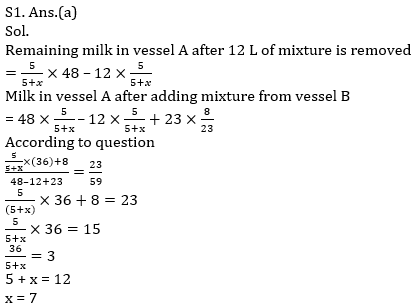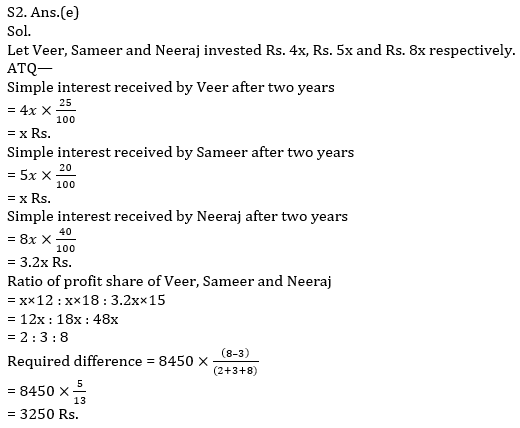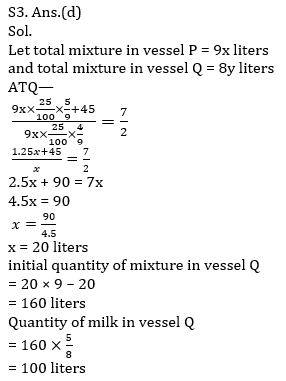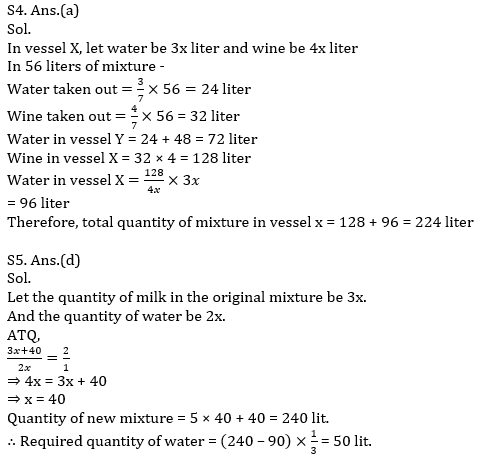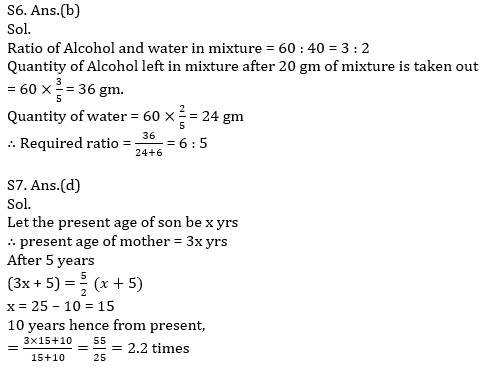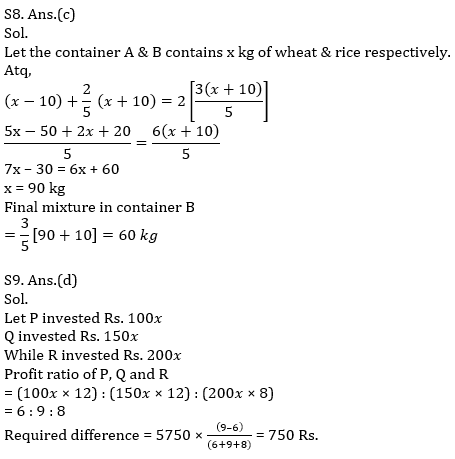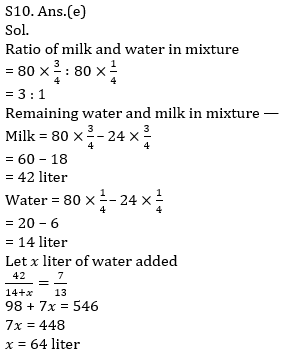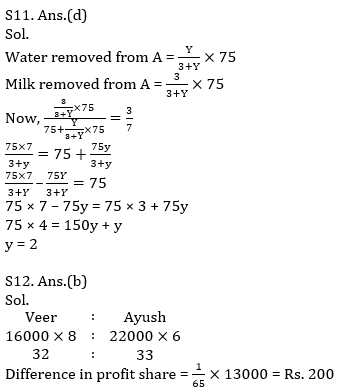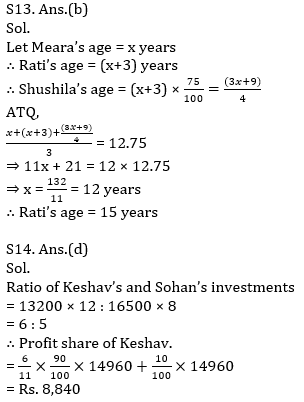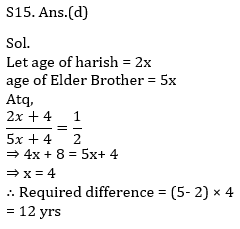×

Thank You, Your details have been submitted we will get back to you.
×
OR

×
OR